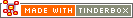Group CodesA family of HTML Export mark-up elements operates on groups of notes. For example:

`^every(child, attribute)^`

returns true if every child of the note has an attribute value of true, and false otherwise.

The possible target groups include:

The operators are:

• ^every^: true if every [group] object's attribute value is true, or if there are no children. Extended syntax: compare explict attribute value
• ^any^: true if any [group] object's attribute value is true; false if there are no children. Extended syntax: compare explict attribute value
• ^count^: counts the number of elements in the group.
• ^sum^: total of all the [group] object's attribute values.
• ^max^: the largest.
• ^min^: smallest of all the [group] object's attribute values.
• ^mean^: computes the arithmetic average of all the [group] object's attribute values.

If the group is empty, the group operator returns the value of the attribute for the current note.

For example, ^any(child,Urgent)^ returns the note's current value of Urgent for notes that have no children. This makes it easier to construct rules, reducing the need for |= and complex ^if^ constructions.

 Up: Export Codes Previous: Export Code Arguments Next: Quick Lists

[Last updated: 14 Dec 2009, using v5.0]